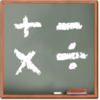現在の価格 ¥ 700（2023/09/29 更新） カテゴリ 教育 評価 （0） （0） 対応デバイス mac
MathBoard® is a highly configurable math app appropriate for all school aged children. Beginning in kindergarten, with simple addition and subtraction problems, through elementary school where learning multiplication and division can be a real challenge. MathBoard will allow you to configure the app to best match the abilities of your individual child/student.

More than just standard drills, MathBoard encourages students to actually solve problems, and not just guess at answers. This is done by providing multiple answer styles, as well as a scratchboard area where problems can be worked out by hand. Students can also turn to MathBoard's Problem Solver for further help. This powerful teaching feature walks students through the steps required to solve addition, subtraction, multiplication, and division equations. Additionally, the included quick reference tables serve as a valuable learning tool.

MathBoard features Math Activities. These activities include Find the Sign, Equality/Inequality, and Match Math (a memory game). Each activity is based upon the current settings level, so the difficulty will vary based on the student’s knowledge.

FEATURES:
- Random equation generation for Addition, Subtraction, Multiplication, Division, Squares, Cubes, and Square Root problems.
- Number ranges are configurable, including the ability to require certain numbers to be in each problem and the ability to omit negative answers.
- Number and order of displayed digits can be limited, allowing for equations to conform to certain learning levels (e.g. 2 digit numbers over 1 digit numbers).
- Generates simple equations, as well as single step algebraic equations. (e.g. 6+x=12; x-8=2; 5x=25).
- Intelligent problem and "wrong answer" generation makes guessing more difficult.
- Includes both multiple choice, as well as, fill in the blank style questions.
- Activities and quizzes can be timed, either as a countdown timer or elapsed time.
- Equation configuration settings can be saved for future use as well as shared with others.
- Multiple student profiles are supported. Includes the ability to save, review, and share the results for activities and quizzes with others.
- Problem Solver will outline the steps needed to solve addition, subtraction, multiplication, and division problems.
- In addition to standard drills, activities are included (Find the Sign, Equality/Inequality and Match Math).
- Quick reference math tables for counting, addition, subtraction, and multiplication.